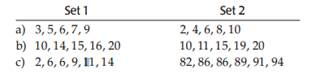### Create an Account

Home / Questions / Standard deviation I For each lettered part a through c examine the two given sets of numb...

# Standard deviation I For each lettered part a through c examine the two given sets of numbers Without doing any calculations decide which set has the larger standard deviation and explain why

Standard deviation I. For each lettered part, a through c, examine the two given sets of numbers. Without doing any calculations, decide which set has the larger standard deviation and explain why. Then check by finding the standard deviations by hand.Apr 26 2020 View more View LessSubscribe To Get Solution